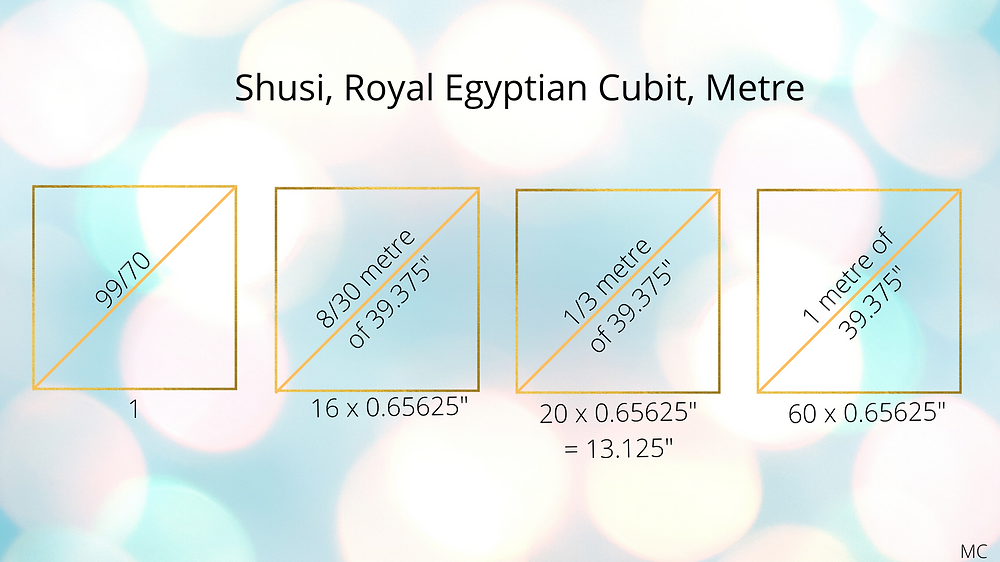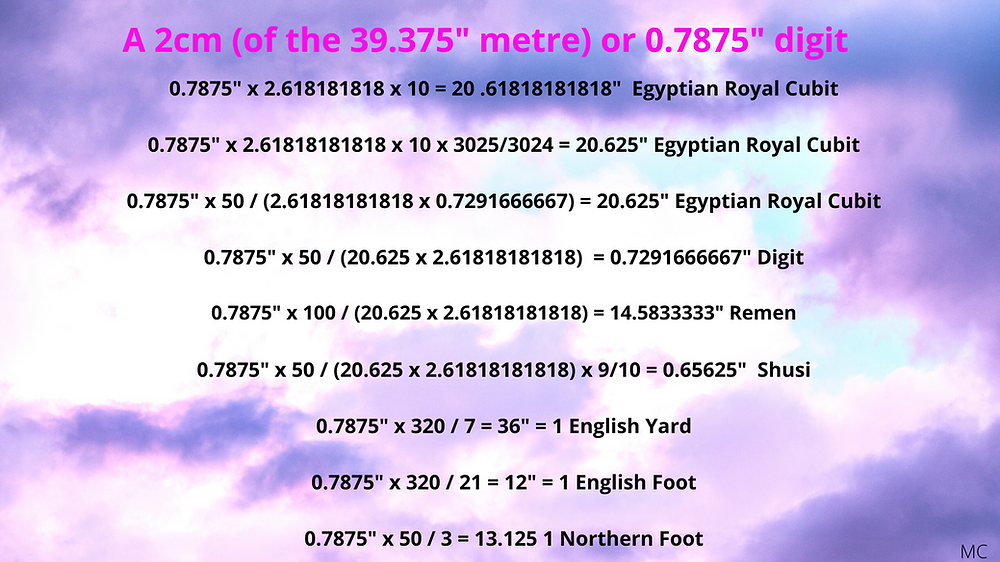Search

# 35. Don't Fear the Metre

Updated: Feb 23Seasons don't fear the metre, nor do the wind, the sun or the rain...

... as Blue Oyster Cult almost sang.

The ancient Egyptians knew this metre very well...

Pages 54-57 in The Temple in Man (Le Temple dans l'Homme), R.A. Schwaller de Lubicz

It seems that in ancient metrology research today, with a few exceptions, no one much likes the metre. It's commonly assumed that the metre is a relatively recent French invention and that using the metre to study ancient measure is anachronistic and misguided. It's also ... well, French - not an attribute those who favour the imperial system necessarily embrace. Worse still, ancient metrologists tend to believe the world's adoption of the metre has put in jeopardy the real star of the show, the English foot, and it's derivatives, which are being increasingly side-lined. Partly because the English foot has been accepted as pre-historic by many researchers into the ancient world, it is all the more valued as a precious and fragile artefact from the ancient world. But what if the French Revolutionaries didn't invent anything, but had consciously re-cycled an ancient and very important unit of measure?

To see this metre at work in ancient measure, it's helpful to focus on the 39.375" value, though there are variations on this, which are equally important, such as the Saxon Wand of 39.6" (see Jim Wakefield's work), and the 39.366" metre (see Berriman's Ancient Metrology)

A 39.375" metre relates to the modern value of 10,000/254 inches as 8001/8000 precisely. A 39.375" metre gives exactly 40,000,000 metres for the earth's circumference, if you accept a 1,575,000,000 inch value.

The cubit is often thought to be the most important unit of prehistoric engineering - though of course there are many values of cubits. Two important ones are given by Berriman in Historical Metrology as 20.625" and 20.61818181818". Most researchers into ancient metrology accept one or the other, or both, as a valid unit in ancient Egypt. The 39.375" metre is central to these lengths.

The evidence for the structure of ancient metrology strongly suggests that all themeasurement systems in use throughout the ancient world formed part of a unified system.

In this paper, John Neal presents the Belgic foot as 1.08 English Feet. It's curious therefore to see this same ratio present between a 0.7875" or 2mm of the 39.375" metre and the 0.729166667" digit, sixteen of which make up an Egyptian or Roman foot.

0.7875 / 1.08 = 0.72916666667

In the same article, Neal writes:

The disadvantages of the metric system as a tool to express ancient measurements are primarily that the module has no provision for a sub-multiple of three, although this is what the metre actually is – a yard from a previous system. It is based, in fact, on the “Belgic”foot. Petrie unwittingly said as much in the course of a treatise upon ancient measures. (16) He stated that had the British adopted the “Belgic” foot instead of the “English” at the time of the statutes instituted by Edward I in 1305, as it was widely used in Britain at that time,
“Then but little adjustment would have been required of us to conform to the metre.”
This statement is the literal truth; consider that there are seventy-five Roman miles,therefore 375,000 Roman feet to the geographic degree. The Belgic foot is the ratio of nine to eight of the Roman so there are 333,333.333 Belgic feet and consequently 111,111.111 Belgic yards and this is the number of metres to the degree. The Belgic foot was used as a geographic measurement in antiquity, and is the basis of Ptolemy’s reckoning of 66 ⅔ miles to the degree. 360,000 ft divided by this number gives the number of feet to the mile as 5,400 each foot of the Belgic 1.08ft to the 5,000ft mile. These are of course Root measures, giving the Root “metre” as 3.24ft, it is more recognisable as a metre if the degree length is takenfrom the average degree at approx 45 degrees the metre length is then close to the accepted 3.2808398 ft. It has this awkward number, 111111.111 to the degree because the French reformers failed to decimalise the degree or the number of hours of the day. They had attempted to do so by devising the grad, but the scientists had finally dug in against such ill-consideredreforms for the simple reason that time and spheres must have a sexagesimal division. Due to the fact that that they could not divide the metre by three, the newly introduced metric system was utterly divorced from the systems that it superseded and this is the reason that ancientmeasurement systems cannot be properly understood in the SI units of centimetres etc.The metric system is a typical committee contrivance of compromise that took the best part of a century to devise and get utterly wrong. Systemic comparisons must be conducted at the foot level, and because the metric foot has been lost then the basic numbers have been lost. Older British metrologists have similarly occluded the study of the field by their fondness for expressing even considerable distances in terms of the inch. This produces the same result, namely the destruction of the founding numbers, which should be expressed as feet.
This term has little to do with the human appendage; in this context foot means basis. For the purposes of comparative metrology even large units such as miles and leagues etc. should be reduced to their basic feet and the comparisons made at that level.

The argument that the metre isn't easily divisible by three seems a strange one to use in order to disprove it's existence in the ancient world. In any case, a unit of 39.3700787402" or 39.375" does not necessarily pre-suppose a decimal or duodecimal system. They are simply units. In fact a third of a 39.375" unit is a Saxon foot of 13.125", or 18 digits of 0.729166667" (though these values also vary).

"The Belgic foot is the ratio of nine to eight of the Roman "

Neal uses slightly different values for his Roman foot but the ratio works with the Roman and Northern feet:

16 x 0.7291666667" = 11.6666667 Roman Foot

Roman foot x 9 / 8 = 13.125"

This is a third of a metre of 39.375", and the Northern foot value that Jim Alison has proposed, which comprises 18 digits or 20 shusi of 0.65626".

It's clear that Neal is no great fan of the modern metre, and he puts his case forward well.

What if, however, we accepted a value of 33.3333 metres as useable and significant, which might perhaps define the width of the Station Stone Rectangle at Stonehenge?

54 is, as John Neal points out, 3/20 x 360. This is the number of digits of 0.729166667" in the 39.375" metre, as well as the 0.733333" digits in the 39.6" Saxon Wand, and the 0.729" digit in the Berriman 39.366" metre.

The 0.729166667" digit x 360 x 3 is in fact 787.5", 20 metres of 39.375", and of course this divided by 20 is 1 metre.

Taking the digit of 0.7291666667, multiplying it by 3 and dividing by 20, it's clear that this is in fact 1/360 metres of 39.365".

This digit is a 360th part of a circle (the circumference of which measures a metre), multiplied by 6.666666... or 20/3. As you can see when it is expressed in digits, with the many sixes after the decimal point, it's two thirds of something, and that something is the 360th part of a metre.

The same applies to the other two above mentioned digits:

0.7333333 x 360 x 3/20 = 39.6

0.729 x 360 x 3/20 = 39.366

I think we need to take a look at the metre and see exactly how it fits in with some of the major measures of antiquity. Here I focus on the 0.729166667" digit and the Egyptian Royal Cubits.3025/3024 is known as the lehmerisma. 864/275 x 3,025/3,024 = 22/7.

22/7 x 7,7760/7,875 = 864/275

The ragisma of 4375/4374 = 70,000/(7,776 x 9), or 9,800/9,801x 3,025/3024.

9,80/9,801 is the Kalisma-Gauss comma ratio.

Together these ratios form an intriguing little group, which Stephen Dail described as a "trinary relationship between (3025/3024) / (4375/4374) = 9801/9800" in a post on GHMB. In this post, in fact, Stephen points out:

" I mentioned in my other topic that curious relationship of the old Meter value being a quadrature multiple of the two Pi products 25/8 or 3.125 x 3.15 = 9.84375 as in inches x 4 = 39.375 in. Meter. And it also happens to be the case that John Michell's Polar Earth radius value of 3960 Mi. x 384/385 = 3949.714286 Mi., is likewise the quadrature product of the two Pi values 22/7 x 864/275 x 400 = 3949.714286 Mi."

This is a very interesting side of the 39.375" metre, connected to two pi approximation values.Perhaps we could say that once we start using the 0.729166667" digit, rather than a 0.729" one, and the 0.65625" Shusi rather than the 0.66" one, we have accepted the importance of the metre, at least the 39.375" one? The same goes with the 20.6181818" cubit, via Phi squared. What the ubiquity of 0.7291666666" and 0.65625" tell us is that the metre is central. That's not to say the units derive from the metre, but that they are intimately connected. The same is true for the imperial system as 6.4 /21 metres (of the 39.375" metre) are a foot.

So naturally a 0.7875" digit should also have nice simple connections to many units.

A 250,000 stadia circumference of the earth, as per Cleomedes, assuming this same measure to be exactly 40,000,000 x 39.375" or 1,575,000,000", is 7,875 x 7,875 x 1,000 metres of 39.375".

To go back to a unit of 30 shusi or 27 digits, that's also 25 x 2 cm or 25 x 0.7875".

And 20 shusi are 18 digits,and also 50/3 x 0.7875".

A 2 cm or 0.7875" unit multiplied by:

• 9,600/7 gives a "U" of 10.8" = 12 x 12 x 12 x 12 / 1,400 digits of 0.729166667" = 230.4 / 14 shusi of 0.65625"

• by 24 gives a quim cubit of 18.9", which is 25.92 digits of 0.72916667", or 28.8 shusi of 0.65625".

• by 27 gives the black cubit of 54 cm = 105 x 12³ x 9 / (8 x 7,000) digits of 0.72916667" = 21.2625" which is 32.4 shusi.

• by 192/7 make a 2"U" unit of 21.6"

• by 28.8 make up a watering cubit ("coudée de l'arroseur") = 57.6 cm = 126 x 12³ / 7,000 digits of 0.729166667" = 22.68", and 34.56 shusi.

• by 32 make up the Hachemi cubit of 64 cm = 25.2" = 34.56 digits = 38.4 shusi

• by 230.4 / 7 make up a 25.92" Royal assyrian cubit = 4,608 / 7 mm = 12⁵ / 7,000 digits = 12 x 12 x 12 x 16 / 700 shusi

• by 320 / 21 are the English foot of 12"

• by 48 make up a Hand cubit ("coudée de la main") = 105 x 12³ / 7,000 digits = 18.9" = 28.8 shusi of 0.65625"

• by 550/21 are the REC of 20.625" = 220/7 shusi of 0.65625" (the English foot being the 20.625" ERC x 32/55, with the common factor beween the English foot and the ERC being 36/7 digits of 0.729166667", this times 55 being 20.625", or times 32 being 12". 36/7 digits are 2/21 metres)

• by 10 x 144/55 are the REC of 20.6181818" = 1,440/55 x 6/5 shusi of 0.65625"

• and by 2,000 / (3 x 36) are the 14.583333" remen (the remen being 4/9 x 2.618181818 / 3.1418181818 metres)